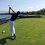In this note, I will discuss how to find the maximum possible integer solutions for x and y in the slight variant of the quadratic Diophantine equation $y^2=a^2x^2+bx+c$. We will focus on the case where $b\neq 2a\sqrt{c}$ since we can factor the RHS into $(ax+c)^2$ and every integer is a solution for $x$.

Begin with the generic quadratic $y=\sqrt{a^2x^2+bx+c}$.

Since $a^2x^2$ is guaranteed a perfect square, $bx+c$ must be a difference of squares. A short proof for this is $y^2=a^2x^2+bx+c\Rightarrow y^2-a^2x^2=bx+c$

This means that the left side can be rearranged to

\begin{aligned} ax+n &=& \sqrt{a^2x^2+bx+c}\\ a^2x^2+2axn+n^2 &=& a^2x^2+bx+c\\ bx-2axn&=&n^2-c\\ x(b-2an)&=&n^2-c\\ x&=&\boxed{\dfrac{n^2-c}{b-2an}}\\ \end{aligned}

NOTE: $n$ is a variable that is an integer.

This is maximized when the denominator is minimized or as the numerator tends infinity. Thus to minimize the denominator, $n\approx \dfrac{b}{2a}$ (look familiar? It's the same as the negation of the vertex of a parabola. Why this is, I have no idea). The $\approx$ sign (it means approximately) is important for two reasons. 1) because $n$ has to be integral for y to be integral and $\dfrac{b}{2a}$ isn't always integral. 2) $n$ can't exactly equal $\dfrac{b}{2a}$ because if it does, then we are dividing by 0 and the world will implode.

NOTE: this is a plug and chug because we need to find an integral value of n that will make the numerator divisible by the denominator.

Now that we have found the maximum value of x, we can plug this in to find y.

$y=\sqrt{a^2x^2+bx+c}$

$y=\sqrt{a^2\left(\dfrac{n^2-c}{b-2an}\right)+b\left(\dfrac{n^2-c}{b-2an}\right)+c}$

$rearranging...$

$\boxed{y=\left|\dfrac{bn-a(n^2+c)}{b-2an}\right|}$

(the long bars denote absolute value.. sorry it looks so bad)

This also further proves that y is maximized when $n$ is as close as possible to $\dfrac{b}{2a}$.

## Practice section

Maximum possible integer square root polynomial 1 (easier)

Maximum possible integer square root polynomial 2 (harder)Note by Trevor Arashiro
6 years, 7 months ago

This discussion board is a place to discuss our Daily Challenges and the math and science related to those challenges. Explanations are more than just a solution — they should explain the steps and thinking strategies that you used to obtain the solution. Comments should further the discussion of math and science.

When posting on Brilliant:

• Use the emojis to react to an explanation, whether you're congratulating a job well done , or just really confused .
• Ask specific questions about the challenge or the steps in somebody's explanation. Well-posed questions can add a lot to the discussion, but posting "I don't understand!" doesn't help anyone.
• Try to contribute something new to the discussion, whether it is an extension, generalization or other idea related to the challenge.

MarkdownAppears as
*italics* or _italics_ italics
**bold** or __bold__ bold
- bulleted- list
• bulleted
• list
1. numbered2. list
1. numbered
2. list
Note: you must add a full line of space before and after lists for them to show up correctly
paragraph 1paragraph 2

paragraph 1

paragraph 2

[example link](https://brilliant.org)example link
> This is a quote
This is a quote
    # I indented these lines
# 4 spaces, and now they show
# up as a code block.

print "hello world"
# I indented these lines
# 4 spaces, and now they show
# up as a code block.

print "hello world"
MathAppears as
Remember to wrap math in $$ ... $$ or $ ... $ to ensure proper formatting.
2 \times 3 $2 \times 3$
2^{34} $2^{34}$
a_{i-1} $a_{i-1}$
\frac{2}{3} $\frac{2}{3}$
\sqrt{2} $\sqrt{2}$
\sum_{i=1}^3 $\sum_{i=1}^3$
\sin \theta $\sin \theta$
\boxed{123} $\boxed{123}$

Sort by:

I have a few questions:

Why did you let $y=ax+n$?

Why is $f(n)=\dfrac{n^2-c}{b-2an}$ maximized at $n\approx \dfrac{b}{2a}$?

- 6 years, 2 months ago

The first is easy to answer. If we look at the second half of the $a^2x^2+bx+c$, then since the first half is already a perfect square, n basically represents the difference between squares. Thus it can be positive or negative. If b and c are positive, it will be positive, if both are negative, n is negative. If one is negative and one is positive, then it gets complicated.

For The second half, it is not maximized there. But that is the largest possible integral value that is achievable by that function. I don't have a "full" proof as I'm very bad at explaining this kind of thing. If you'd like me to I could try to type up a full explicit proof for this.

Which also reminds me. I have a proof of why n must be integral for x to be integral. I could add that if you'd like me to.

- 6 years, 2 months ago

How did you wrote $y =ax+n$?

And there is $x^{2}$ missing in the same expression.

- 6 years, 7 months ago

I don't know what you mean by missing a $x^2$

But $y=ax+n$ because $a^2x^2$ is guaranteed a perfect square, so $bx+c$ must be a difference between two squares. Assuming that $bx+c\neq 0$, n is just an arbitrary value that is the difference between $a^2x^2$ and $y^2$. You could guess many numbers for the Max value of x to find a perfect square value for $a^2x^2+bx+c$, but you would never know when to stop. This method allows you to check for values of n and know when you find the max value for x (since the denominator should be minimized).

- 6 years, 7 months agothere it is

- 6 years, 7 months ago

ah, thanks, missed that

- 6 years, 7 months ago